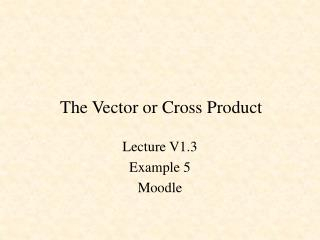DownloadDownload PresentationThe Vector or Cross Product

# The Vector or Cross Product

Download Presentation## The Vector or Cross Product

- - - - - - - - - - - - - - - - - - - - - - - - - - - E N D - - - - - - - - - - - - - - - - - - - - - - - - - - -
##### Presentation Transcript

1. The Vector or Cross Product Lecture V1.3 Example 5 Moodle

2. Definition of the Cross Product commutative law does NOT hold

3. Distributive Law

4. Cross Product and Vector Components

5. Associative Law Does NOT, in general, hold for the cross product

6. Cross Product Geometric Properties Area of parallelogram is h |A| =

7. Cross Product Geometric Properties The volume of the parallelepiped is equal to (area of parallelogram formed by A and B) (height h)

8. Scalar Triple Product

9. Matlab Example 1 and Given the vectors and find = 14j + 7k

10. Matlab Example 1 and Given the vectors and find >> A = [1 -2 4] A = 1 -2 4 >> B = [3 1 -2] B = 3 1 -2 >> C = cross(A,B) C = 0 14 7 >> magC = norm(C) magC = 15.6525 >> = 14j + 7k

11. Matlab Example 2 and Given the vectors find the angle between A and B using >> A = [1 -2 4] A = 1 -2 4 >> B = [3 1 -2] B = 3 1 -2 >> phi = 180 - (asin(norm(cross(A,B))/(norm(A)*norm(B))))*180/pi phi = 114.0948 >> .